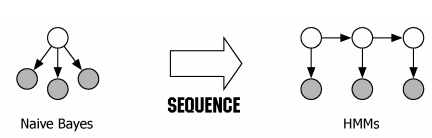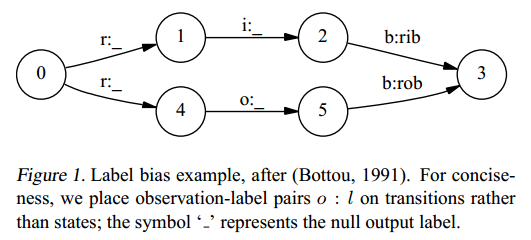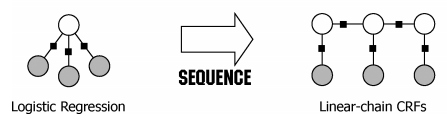# 从HMM到MEMM再到CRF

## 1. HMM

HMM是一种生成式模型，它的理论基础是朴素贝叶斯，本质上就类似于我们将朴素贝叶斯在单样本分类问题上的应用推广到序列样本分类问题上。$P(y|x)=\frac{P(x|y)*P(y)}{P(x)}$

$P(y_1^n|x_1^n)=\frac{P(x_1^n|y_1^n)*P(y_1^n)}{P(x_1^n)}$

我们以词性标注这一序列化分类问题进行后面的讲解。对于一个给定的句子$x_i^n=(x_1,x_2,...,x_n)$，我们的目的是为其找到一个得分最高的标签序列$\hat{y}_1^n=({\hat{y}_1,\hat{y}_2,...,\hat{y}_n})$，则该问题转化为:
$\hat{y}_1^n=\arg \limits_{y_1^n} max \frac{P(x_1^n|y_1^n)*P(y_1^n)}{P(x_1^n)}$

$\hat{y}_1^n=\arg \limits_{y_1^n} maxP(x_1^n|y_1^n)*P(y_1^n)$

• 齐次马尔可夫性假设，即假设隐藏的马尔科夫链在任意时刻$t$的状态只依赖于其前一时刻的状态，与其他时刻的状态及观测无关，也与时刻$t$观测无关。
• 观测独立性假设，即假设任意时刻的观测只依赖于该时刻的马尔科夫链的状态，与其他观测及状态无关。

$P(y_1^n)=P(y_1)*P(y_2|y_1)*P(y_3|y_1,y_2)*...*P(y_n|y_1^{n-1})$

$P(y_1^n)=P(y1)*P(y_2|y_1)*P(y_3|y_2)*...*P(y_n|y_{n-1})$

$P(x_1^n|y_1^n)=P(x_1|y_1)*P(x_2|y_2)*...*P(x_n|y_n)$

$\hat{y}_1^n=\arg \limits_{y_1^n} max\prod_{t=1}^{n}P(x_t|y_t)*P(y_t|y_{t-1})$

• $X$表示的是可观测序列集合，在词性标注中对于词汇表
• $Y$表示的是状态集合，在词性标注中对应词性集合
• $A$表示状态转移概率，从一个状态转化到另一个状态，对应$P(y_t|y_{t-1})$
• $B$表示发射概率，由状态预测观测值的概率，对应$P(x_t|y_t)$
• $\pi$表示初始状态的概率分布，对应$P(y_1)$

$\nu_t(j)=\max_i^n \nu_{t-1}(i)*a_{ij}*b_j(x_t)\;\;1\leq j \leq n, 1<t<T$

HMM试图去拟合$X,Y$joint likelihood(联合分布$P(X,Y)$)，所以它是一种生成式模型，它拥有以下几个缺陷：

• 在很多序列化标注任务中，尤其当不能枚举观测值时，需要用大量的特征来刻画观察序列。如在文本识别中识别一个未见的公司名字时，除了传统的单词识别方法以外，还需要用到很多特征信息，如大写字母、结尾词、词性等信息。也就是，我们需要用特征对观测值进行参数化，而HMM中直接利用观测值本身。
• 在很多自然语言处理任务中，需要解决的问题是在已知观测序列的情况下求解状态序列，HMM采用的生成式的联合概率分布$P(x_1^n,y_1^n)$来求解条件概率$P(y_1^n|x_1^n)$，这种方法不适合处理很多特征描述观测序列的情况。为此MEMM直接采用条件概率模型$P(y_1^n|x_1^n)$

## 2.MEMM

最大熵马尔科夫模型利用判别式模型的特点(建议先了解最大熵模型)，直接对每一个时刻的状态建立一个分类器，然后将所有的分类器的概率值连乘起来。为了实现是对整个序列进行的分类，在每个时刻t时，它的特征不仅来自当前观测值$x_t$，而且还来自前一状态值$y_{t-1}$。所以MEMM中，给定观测序列$x_1^n$,某个状态序列$y_1^n$的概率是：
$P(y_1^n|x_1^n)=\prod_{t=1}^nP(y_t|y_{t-1}, x_t)\;\;公式2.1$

$P(y_t=y^*|y_{t-1}=y\prime,x_t)=\frac{1}{Z(x_t, y\prime)}exp \left( \sum_a \lambda_af_a(x_t, y\prime, y^*) \right)\;\;公式2.2$

$Z(x_t,y\prime)=\sum_yexp\left( \sum_a\lambda_af_a(x_t,y\prime,y) \right)$

使用维特比算法进行解码时，MEMM对应的公式为:
$\nu_t(j)=\max_i^n \nu_{t-1}(i)*P(y_j|y_i,x_t)\;\;1\leq j \leq n, 1<t<T$

通过上面的公式，你会发现最大熵模型在每一个时刻，针对不同的前一状态$y\prime$进行归一化操作，这是一种局部的归一化操作，会存在标签偏置问题。以CRF论文中原始的例子为例：它会造成什么后果呢？假设训练集现在有3个rib和1个rob，当我们在测试阶段，遇到词rob，它会被解码成什么状态序列呢？答案是$(0\to1 \to 2 \to 3)$！你可以套公式2.1试一试，因为$P(1|0,"r") > P(4|0,"r")$ , $P(2|1,"o") = P(5|4,"0")=1$,$P(3|2,"b") > P(3|5,"b")$

那么问题出在哪里呢？因为MEMM中在每一时刻t，都在前一时刻某状态$y\prime$下做了局部的归一化操作，如何解决这种标签偏置问题呢？在CRF中并不是对每个时刻都进行一次分类，而是直接对整个序列进行分类，做一个全局的归一化操作。

## 3.CRF

CRF是一种判别式模型，它的理论基础是逻辑回归。这里我们将从单样本二分类问题扩展到单样本多分类问题，最后延伸到序列样本多分类问题。

在单样本二分类问题中使用逻辑回归，假设给定输入$\bold{x}$，定义其分类概率为：
$h_{\theta}(\bold{x})=g(\theta^T\bold{x})=\frac{1}{1+e^{-\theta^T\bold{x}}}$

在单样本多分类问题中使用softmax分类器，假设存在样本$x$，其被分成标签$y=y^j$的概率为：
$P(y=y^j|x)=\frac{1}{Z(x)}exp\left( \sum_{k=1}^m\lambda_kf_k(x,y^j) \right)$

$Z(x)=\sum_{y}exp\left( \sum_{k=1}^m\lambda_kf_k(x,y) \right)$条件随机场CRF是逻辑回归的一种扩展，将单样本多分类问题拓展到序列化样本多分类问题。它不像MEMM那样由一连串的分类器累积而成，而是直接将整个序列化分类当成一个整体的分类任务，进行全局的归一化。假设给定输入$x_1^n$，标签序列$y_1^n$的概率为:
$P(y_1^n|x_1^n)=\frac{1}{Z(x_1^n)}exp\left( \sum_{t=1}^n\left( \sum_{k=1}^m\lambda_kf_k(x_t,y_t) + \sum_{l=1}^s \mu_lg_{l}(y_{t-1},y_t)\right) \right)$

$\sum_{\hat{y}\in Y}exp\left( \sum_{t=1}^n\left( \sum_{k=1}^m\lambda_kf_k(x_t,\hat{y}_t) + \sum_{l=1}^s \mu_lg_{l}(\hat{y}_{t-1},\hat{y}_t)\right) \right)$

更多的关于线性条件随机场的请参考这里